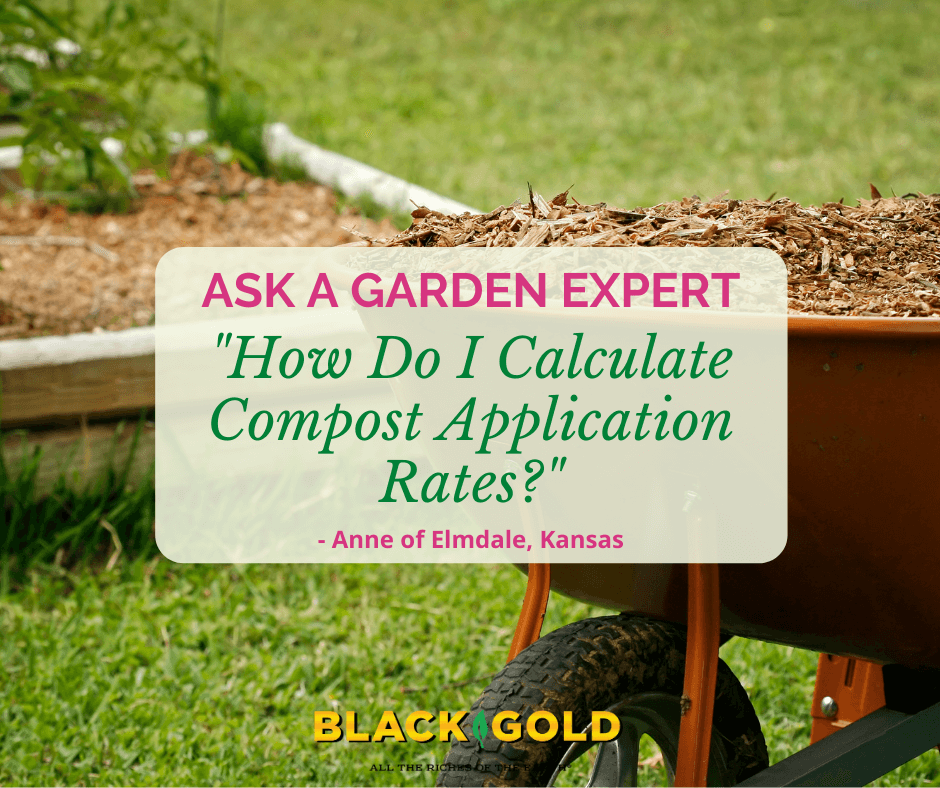# Articles

## How Do I Calculate Compost Application Rates?“How do I calculate compost application rates to yards?  I am trying to figure how much to order.” Question from Anne of Elmdale, Kansas

Answer: To determine how much compost, topsoil, or mulch to apply to beds, use the formula below along with a conversion table and links to other helpful sources–most notably, a great article for easily calculating the square footage of beds of various shapes.

## Amendment Application Formula for Beds

For square or rectangular beds, multiply the bed’s length by its width to get square footage (L *W= ft2). If you wish to lay mulch 2-inches deep, then have a look at the table below to get the corresponding square footage covered by 1 cubic yard of compost, mulch, or peat moss. Then divide the square footage in the table that matches the 2-inch depth.